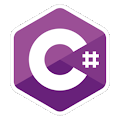Programming Course in C# ¡Free!

# Binary

Proposed exercise

Create a program that asks the user for a decimal number and displays its equivalent in binary form. It should be repeated until the user enters the word "end." You must not use "ToString", but succesive divisions.

Output

Solution

```using System;
public class Binary
{
public static void Main()
{
string result;
string text;

do
{
Console.Write("Number to convert: ");

if (text != "end")
{
int n = Convert.ToInt32( text );
result = "";

while (n > 1)
{
result = Convert.ToString( n % 2 ) + result;
n /= 2;
}

result = Convert.ToString(n) + result;
Console.WriteLine("Binary: {0}", result);         }
}
while (text != "end");
}
}

```